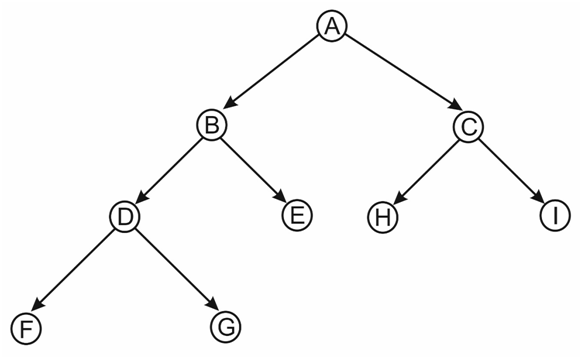# Delete a Tree

Time Complexity : O(n)
Space Complexity :O(1)

Here we use, Post-Order traversal method to delete the tree nodes. Because, before deleting the parent node we should delete children nodes.

### ExampleFigure : Binary tree
If we apply Treedelete(A),
The order of deletion of nodes is: F, G, D, E, B, H, I, C,

## Algorithm

Step 1 : we create a delete tree function. which deletes any sub-tree you want to delete.
Step 2 : In this we use post-order traversal recursive method, but instead of printing the node when we visit it, we delete it.
a)     We use free(node) to delete the node.
b)    While deleting we print that we are deleting the particular node.
Step 3 : we make the root null after deleting the whole tree. Because, if user try to access the values without making to  null it causes problems using root pointer.

## C++ Program

```/* code for deletion of a tree*/
#include <bits/stdc++.h>
#include <stdio.h>
#include <stdlib.h>
using namespace std;

/* implementation of the binary tree with pointers to left child node and right child node*/
struct node
{
char data;
struct node* left;
struct node* right;
};
/*In this function we are creating a node with the given data and null left and right pointers  */
struct node* CreateNewNode(char data)
{
struct node* node = (struct node*)malloc(sizeof(struct node));
node->data = data;
node->left = NULL;
node->right = NULL;
return(node);
}
/*This is the recursive function for Postorder traversal*/
void PostorderTraversal(struct node* node)
{
if (node == NULL)
return;
PostorderTraversal(node->left);/*Recrsion on Left sub-tree*/
PostorderTraversal(node->right); /*Recursion on Right sub-tree*/
printf("%c ", node->data); /*printing data of the node*/

}
/*Deleting Tree in Post-Order Traversal method */
void TreeDelete(struct node* node)
{
if (node == NULL){
return;
}
TreeDelete(node->left);//deleting left subtree
TreeDelete(node->right);//deleting right subtree
printf("\n Deleting node: %c", node->data);
free(node);//deleting the node
}
/*Main function*/
int main()
{
/*Here we are creating a tree by inserting elements*/
struct node *root  = CreateNewNode('A');
root->left             = CreateNewNode('B');
root->right           = CreateNewNode('C');
root->right->left     = CreateNewNode('H');
root->right->right      = CreateNewNode('I');
root->left->left     = CreateNewNode('D');
root->left->left->left     = CreateNewNode('F');
root->left->left->right     = CreateNewNode('G');
root->left->right   = CreateNewNode('E');

cout<<"Post-order traversal of the given input binary tree is :";
/*Printing the tree using Post-order Traversal*/
PostorderTraversal(root);
cout<<"\n";
/*deleting the whole tree*/
cout<< " Deleting Tree.......! "  ;
TreeDelete(root);
root = NULL;
cout<<"\n";
cout<< " Tree Deleted!! "  ;
return 0;
}```

Translate »